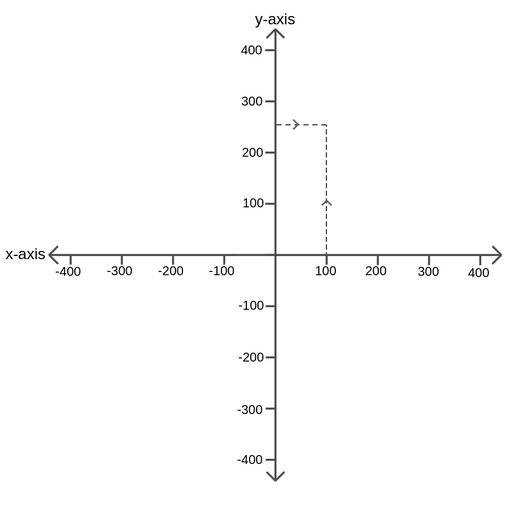A Cartesian coordinate plane is a system which uses one or more numbers, or coordinates, to uniquely determine the position of a point or other geometric elements.

In a two dimensional system, there should be two perpendicular lines; the line with the horizontal direction is commonly called x-axis and the line with the vertical direction is commonly called y-axis. The increments of each axis and the lowest and highest value, define the scale of the axis.

To find the coordinates of a point in the Cartesian coordinate system you begin at the point of interest and follow a vertical line either up or down to the x-axis. This is the x-coordinate. And then do the same but following a horizontal line to find the y-coordinate. So, you determine how far up the y-axis and how far along the x-axis the point is. The x and y axes cross at the point (0,0). Usually, Cartesian coordinates are put in parentheses with the x-coordinate first and then the y-coordinate. The figure below shows an example of how to express the coordinates.Figure: The Cartesian coordinates of the point (100,250)